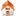# Solving or resolving global tomographic models with spherical wavelets, and the scale and sparsity of seismic heterogeneity

## Frederik J Simons1, Ignace Loris2, Guust Nolet3, Ingrid C. Daubechies4, Sergey Voronin4, J. Stephen Judd4, Philip A. Vetter4, Jean Charléty3 & Cédric Vonesch4

### 4 Program in Applied and Computational Mathematics Princeton University Princeton NJ 08544, USA

Geophys. J. Int., 187 (2), 969–988, 2011, doi: 10.1111/j.1365-246X.2011.05190.x
 Reprint Related work Software Figure software Publications

#### Figures

1. Figure 01 Geometry of the cubed sphere in a three-dimensional view
2. Figure 02 Geometry of the cubed sphere in a two-dimensional view, and nomenclature
3. Figure 03 The looks of the D4 wavelets as they are being used on the cubed sphere
4. Figure 04 The looks of the CDF4.2 wavelets as they are being used on the cubed sphere
5. Figure 05 Preconditioned interval wavelet transforms on the faces of the cubed sphere: thresholding, compression, and coefficient statistics
6. Figure 06A Analysis of wavelet sparsity in the seismic model of Montelli using preconditioned D4 wavelets on the cubed sphere
7. Figure 06B Analysis of wavelet sparsity in the seismic model of Ritsema using preconditioned D4 wavelets on the cubed sphere
8. Figure 07A Analysis of the scale and sparsity in the seismic model of Montelli using preconditioned D4 wavelets on the cubed sphere
9. Figure 07B Analysis of the scale and sparsity in the seismic model of Ritsema using preconditioned D4 wavelets on the cubed sphere
10. Figure 08 Scale lengths of seismic heterogeneity in the seismic models of Montelli and Ritsema, broken down per chunk
11. Figure 09 Correlation between velocity anomalies in the seismic models of Montelli and Ritsema
12. Figure 12 Performance of the algorithm on a semi-realistic synthetic test

Frederik Simons